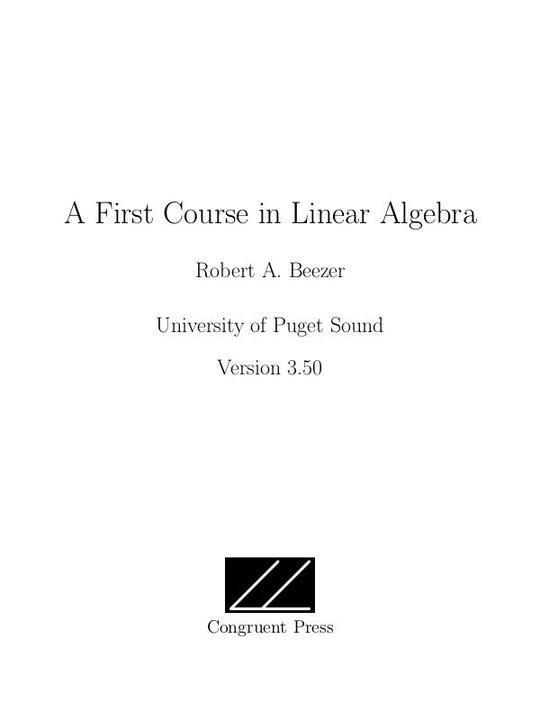Free

# A First Course in Linear Algebra

By Robert A. Beezer
Free
Book Description

This book is a well-organized text with carefully constructed examples, a full quota of exercises with solutions, and an emphasis that is algebraic rather than geometric. The book is Sage-enabled with approximately 90 examples of Sage code spread throughout. The book sections can be loaded into Sage as worksheets so that the code can be evaluated immediately; however, it is not necessary to use Sage in order to make use of this textbook.

The HTML version is a fully featured electronic text with cross references that expand in place. It works well on an iPad or any device with Internet access. The Sage examples are executed by the Sage single cell server over the Internet, and students can experiment with their own examples as they read without needing to install Sage or log into a Sage server. Most students will prefer this version over both the pdf and print versions.

As stated in the preface of the book, the dual aims are “to teach the fundamental concepts and techniques of matrix algebra and abstract vector spaces, and to teach the techniques associated with understanding the definitions and theorems forming a coherent area of mathematics.”

Verion 3.50 (spring 2016) is archived here. Online and Hardcover versions are available via the author's website.

• Preface
• Acknowledgements
• Systems of Linear Equations
• What is Linear Algebra?
• Solving Systems of Linear Equations
• Reduced Row-Echelon Form
• Types of Solution Sets
• Homogeneous Systems of Equations
• Nonsingular Matrices
• Vectors
• Vector Operations
• Linear Combinations
• Spanning Sets
• Linear Independence
• Linear Dependence and Spans
• Orthogonality
• Matrices
• Matrix Operations
• Matrix Multiplication
• Matrix Inverses and Systems of Linear Equations
• Matrix Inverses and Nonsingular Matrices
• Column and Row Spaces
• Four Subsets
• Vector Spaces
• Vector Spaces
• Subspaces
• Linear Independence and Spanning Sets
• Bases
• Dimension
• Properties of Dimension
• Determinants
• Determinant of a Matrix
• Properties of Determinants of Matrices
• Eigenvalues
• Eigenvalues and Eigenvectors
• Properties of Eigenvalues and Eigenvectors
• Similarity and Diagonalization
• Linear Transformations
• Linear Transformations
• Injective Linear Transformations
• Surjective Linear Transformations
• Invertible Linear Transformations
• Representations
• Vector Representations
• Matrix Representations
• Change of Basis
• Orthonormal Diagonalization
• Preliminaries
• Complex Number Operations
• Sets
• Reference
• Proof Techniques
• Archetypes
• Definitions
• Theorems
• Notation
No review for this book yet, be the first to review.
No comment for this book yet, be the first to comment
Also Available On
Categories
Curated Lists
• #### Free Machine Learning Books

11 Books

Pattern Recognition and Machine Learning (Information Science and Statistics)
by Christopher M. Bishop
Data mining
by I. H. Witten
The Elements of Statistical Learning: Data Mining, Inference, and Prediction
by Various
See more...
• #### Free Chemistry Textbooks

9 Books

CK-12 Chemistry
by Various
Concept Development Studies in Chemistry
by John Hutchinson
An Introduction to Chemistry - Atoms First
by Mark Bishop
See more...
• #### Free Mathematics Textbooks

21 Books

Microsoft Word - How to Use Advanced Algebra II.doc
by Jonathan Emmons
Advanced Algebra II: Activities and Homework
by Kenny Felder
de2de
by
See more...
• #### Free Children Books

38 Books

The Sun Who Lost His Way
by
Tania is a Detective
by Kanika G
Firenze_s-Light
by
See more...
• #### Free Java Books

10 Books

Java 3D Programming
by Daniel Selman
The Java EE 6 Tutorial
by Oracle Corporation
JavaKid811
by
See more...